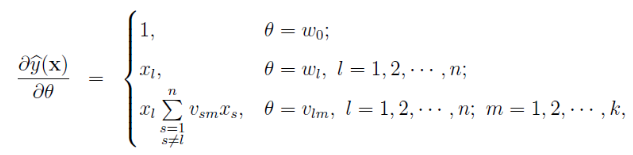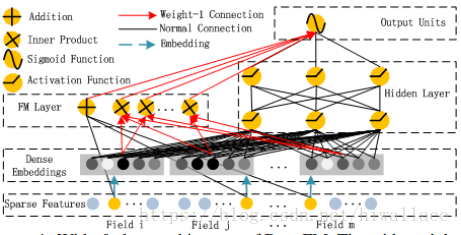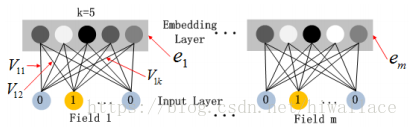FM分解机及其变种(FFM、DeepFM)原理详解

FM算法

一、背景

FM(Factorization Machine)主要是为了解决数据稀疏的情况下，特征怎样组合的问题。

$y=w_0+\sum^n_{i=1}w_ix_i$

$y=w_0+\sum^n_{i=1}w_ix_i+\sum^{n-1}_{i=1}\sum^n_{j=i+1}w_{ij}x_ix_j$

二、目标函数

FM的思路为，在多项式模型的基础上，通过矩阵分解的方法，为了求解$w_{ij}$，为每一个特征分量xi引入辅助向量$V_i=(v_{i1},v_{i2},...,v_{ik})$，然后用$v_iv_j^T$$w_{ij}$进行求解。

$y=w_0+\sum^n_{i=1}w_ix_i+\sum^{n-1}_{i=1}\sum^n_{j=i+1}(v_iv_j^T)x_ix_j$

$\sum^{n-1}_{i=1}\sum^n_{j=i+1}(v_iv_j^T)x_ix_j = \frac{1}{2}\sum^k_{l=1}[(\sum^n_{i=1}(v_{il}x_{i})^2-\sum^n_{i=1}v_{il}^2x_{i}^2]$
FM的复杂度为$O(kn^2)$，通过上述等式的改写，可将复杂度降为线性的$O(kn)$。因此，FM可以在线性时间对新样本做出预测，复杂度和LR模型一样，且效果提升不少。

三、训练算法

FM可用于各种预测任务，如回归、分类、排序等。

$y=g_\theta(x)+\theta h_\theta(x)$四、FM 对比 其他算法

FM对比SVM

• SVM的二元特征交叉参数是独立的，而FM的二元特征交叉参数是两个k维的向量vi、vj，交叉参数就不是独立的，而是相互影响的。
• FM可以在原始形式下进行优化学习，而基于kernel的非线性SVM通常需要在对偶形式下进行
• FM的模型预测是与训练样本独立，而SVM则与部分训练样本有关，即支持向量

总结

FM优点：

• FM模型可以在非常稀疏的数据中进行合理的参数轨迹，而SVM做不到这点。
• FM模型的复杂度是线性的，优化效果很好，而且不需要像SVM一样依赖于支持向量。
• FM是一个通用模型，它可以用于任何特征值为实值的情况。而其他因式分解模型只能用于一些输入数据比较固定的情况。

FFM算法（Field-aware Factorization Machine）

FFM在FM的基础上进一步改进，在模型中引入类别的概念，即field。将同一个field的特征单独进行one-hot，因此在FFM中，每一维特征都会针对其他特征的每个field，分别学习一个隐变量，该隐变量不仅与特征相关，也与field相关

$y(X)=w_0+\sum_{i=1}^nw_ix_i+\sum_{i=1}^n<V_{i,f_j},V_{j,f_i}>x_ix_j$

DeepFM

FM通过对于每一位特征的隐变量内积来提取特征组合，最后的结果也不错，虽然理论上FM可以对高阶特征组合进行建模，但实际上因为计算复杂度原因，一般都只用到了二阶特征组合。对于高阶特征组合来说，我们很自然想到多层神经网络DNN。DeepFM目的是同时学习低阶和高阶的特征交叉，主要由FM和DNN两部分组成，底部共享同样的输入。模型可以表示为：
$y=sigmoid(y_{FM}+y_{DNN})$
FM部分

$y_{FM}=<w,x>+\sum_{i=1}^d\sum_{j=i+1}^d<V_i,V_j>x_i⋅x_j$

Deep部分1） 尽管不同field的输入长度不同，但是embedding之后向量的长度均为k
2） 在FM中得到的隐变量$V_{ik}$现在作为嵌入层网络的权重

$a^{(l+1)}=σ(W^{(l)}a^{(l)}+b^{(l)})$

$y_{DNN}=W^{|H|+1}⋅a^{|H|}+b^{|H|+1}$
|H|为隐藏层层数。**勘误**： Multilinearity部分最后一句，应该是gθ(x)则利用...来计算。 **注释**： 对(2.6)改写，将复杂度降为线性的O(kn)的推导，第一步即求矩阵上三角，相当于求整个矩阵减去对角线再除以2。